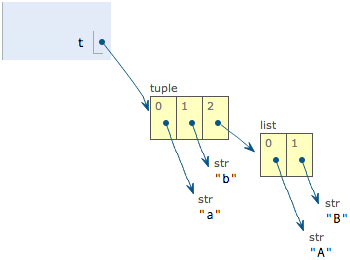# 使用list和tuple

## list

Python内置的一种数据类型是列表：list。list是一种有序的集合，可以随时添加和删除其中的元素。

``````>>> classmates = ['Michael', 'Bob', 'Tracy']
>>> classmates
['Michael', 'Bob', 'Tracy']
``````

``````>>> len(classmates)
3
``````

``````>>> classmates
'Michael'
>>> classmates
'Bob'
>>> classmates
'Tracy'
>>> classmates
Traceback (most recent call last):
File "<stdin>", line 1, in <module>
IndexError: list index out of range
``````

``````>>> classmates[-1]
'Tracy'
``````

``````>>> classmates[-2]
'Bob'
>>> classmates[-3]
'Michael'
>>> classmates[-4]
Traceback (most recent call last):
File "<stdin>", line 1, in <module>
IndexError: list index out of range
``````

list是一个可变的有序表，所以，可以往list中追加元素到末尾：

``````>>> classmates.append('Adam')
>>> classmates
``````

``````>>> classmates.insert(1, 'Jack')
>>> classmates
``````

``````>>> classmates.pop()
>>> classmates
['Michael', 'Jack', 'Bob', 'Tracy']
``````

``````>>> classmates.pop(1)
'Jack'
>>> classmates
['Michael', 'Bob', 'Tracy']
``````

``````>>> classmates = 'Sarah'
>>> classmates
['Michael', 'Sarah', 'Tracy']
``````

list里面的元素的数据类型也可以不同，比如：

``````>>> L = ['Apple', 123, True]
``````

list元素也可以是另一个list，比如：

``````>>> s = ['python', 'java', ['asp', 'php'], 'scheme']
>>> len(s)
4
``````

``````>>> p = ['asp', 'php']
>>> s = ['python', 'java', p, 'scheme']
``````

``````>>> L = []
>>> len(L)
0
``````

## tuple

``````>>> classmates = ('Michael', 'Bob', 'Tracy')
``````

tuple的陷阱：当你定义一个tuple时，在定义的时候，tuple的元素就必须被确定下来，比如：

``````>>> t = (1, 2)
>>> t
(1, 2)
``````

``````>>> t = ()
>>> t
()
``````

``````>>> t = (1)
>>> t
1
``````

``````>>> t = (1,)
>>> t
(1,)
``````

Python在显示只有1个元素的tuple时，也会加一个逗号`,`，以免你误解成数学计算意义上的括号。

``````>>> t = ('a', 'b', ['A', 'B'])
>>> t = 'X'
>>> t = 'Y'
>>> t
('a', 'b', ['X', 'Y'])
``````## 练习

``````# -*- coding: utf-8 -*-

L = [
['Java', 'Python', 'Ruby', 'PHP'],
]

# 打印Apple:
print(?)
# 打印Python:
print(?)
# 打印Lisa:
print(?)
``````

## 小结

list和tuple是Python内置的有序集合，一个可变，一个不可变。根据需要来选择使用它们。

the_list.py

the_tuple.py